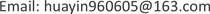——基于门槛回归分析1. 引言

1912年，熊·彼得  在其著作《经济发展理论》一书中，提出了创新理论这一独特的经济理论，人们越发的关注起了技术创新对于经济增长到底起到了怎么样的作用。我国期望在2020年建设成为创新型国家，因此，对于政府R & D资助的大中型企业的创新的影响，必定要将其置于转型经济中科技创新改革的制度背景之下  。

2. 文献综述

3. 实证分析3.1. 模型设定与变量说明

y i t = f ( K , L ) = A K α L β e μ i + ε i t (1)

y i t = f ( K , L ) = A G O V i t α 1 S i t α 2 F i t α 3 O i t α 4 e u i + ε i t (2)

ln ( y i t ) = δ + α 1 ln G O V i t + α 2 ln S i t + α 3 ln F i t + α 4 ln O i t + β ln L i t + θ ′ X i t + μ i + ε i t (3)

3.2. 数据来源

3.3. 实证分析3.3.1. 政府R & D资助对企业技术创新的有效性检验

Impact of government R & D funding on corporate technology innovatio

lngov0.176214***0.0800652.200.0290.176214***
lnl0.554588***0.1206494.600.0000.554588***
lnsize0.035385***0.0074554.750.0000.035385***
pro−5.25658***0.71378−7.360.000−5.25658***
lns0.0959640.0529781.810.0720.095964
lnf0.0131850.017280.760.4470.013185
lno-0.003180.039837−0.080.936−0.00318
c7.682896***1.2548556.120.0007.682896***
hausman检验c h i 2 ( 7 ) = ( b − B ) ′ [ ( V b − V B ) ( − 1 ) ] ( b − B ) = 90.53 Prob > chi2 = 0.0000

3.3.2. 政府R & D资助的门槛分析

ln ( y i t ) = δ + β ln L i t + λ 1 ln G O V i t × I ( q i t ≤ τ ) + λ 2 ln G O V i t × I ( q i t > τ ) + θ ′ X i t + μ i + ε i t (4)

Threshold effect self-sampling tes

Threshold estimation result

Threshold model estimation result

lngov_10.220 (1.87)***0.18 (2.20)***
lngov_20.312 (2.31)***-
lngov_30.264 (1.98)**-
lnl0.567 (5.02)***0.55 (4.60)***
lnsize0.012 (1.61)*0.04 (1.81)***
Pro1.696 (2.56)**−5.26 (−7.36)***

4. 结论及政策建议4.1. 结论

4.2. 政策建议

4.3. 不足